# Python Pandas For Machine Learning

Pandas is a python library used to manipulate data and as we know machine learning is all about training with data, hence it is one the most used python library in many machine learning algorithms, Whether it be the indexing of data or Vectorization of data, pandas library is the fast and effective library to implement all the data manipulation techniques. Let’s see some of the most used pandas library function with the help of code:-

## Indexing Using Pandas Library

Indexing is the basic technique that we can implement using pandas, Let’s see the steps involved in it:-

1. Importing library
2. Creating a list of numeric data
3. Using the library for indexing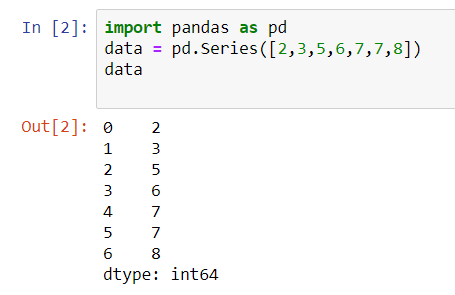We can manipulate indexing with pandas, let’s see how-:

``````Marks = pd.Series(['60','65','67','73'], index=['C++', 'Data structure', 'Python', 'Java'])
Marks``````## Argmax

Argmax function is used to find out the index with the maximum argument, let’s see-: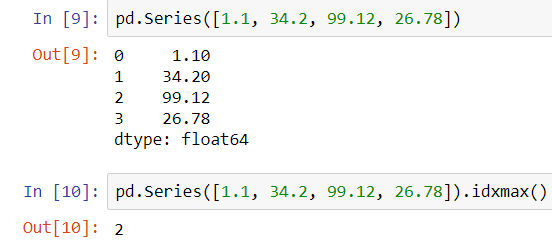## Vectorization Operation Using Python Pandas

We can perform the vectorized operation with pandas, let’s see how:-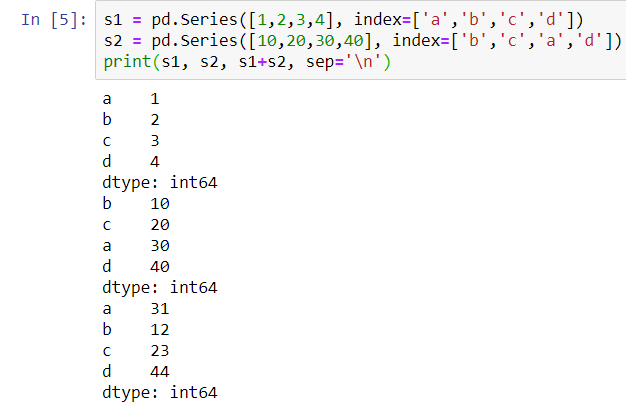## Abbreviation

Abbreviation function is quite an interesting one, let’s see how it works with an example-:

``````countries = pd.Series(["India", "Canada", "Australia", "Denmark"])
countries.apply(abbreviate)``````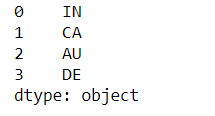## Describe Function

Describe Function is used to describe various insights about the given data, let’s see with the help of an example:-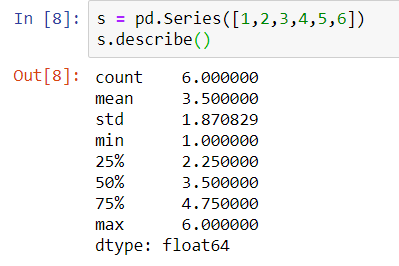## Python Pandas DataFrame

Creating a Dataframe with pandas, This function of the panda’s library takes many rows and columns, Let’s figure it out with the help of an example and see how it works.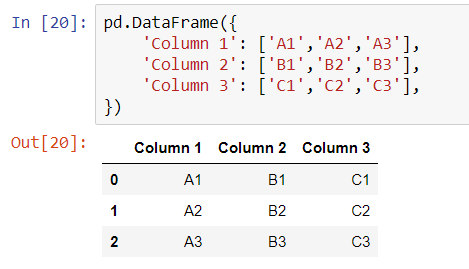## Read CSV files with Pandas

Pandas is able to read large CSV files and that too in a fraction of time, let’s see how it reads it:-

``ds = pd.read_csv('../datasets/titanic.csv')``

You can get more information about the CSV data, with the help of .info() function, let’s seeI hope you got the intuition behind using this library for machine learning, To see its an implementation in machine learning algorithms such as Decision Tree and Random forest, and to learn more about Machine Learning and Deep Learning using Python, stay connected with us.### tanesh

Founder Of Aipoint, A very creative machine learning researcher that loves playing with the data.